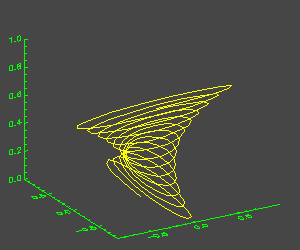# Projecting a Particle in 3D Space

QUESTION: How can I project and display a particle trajectory in 3D space?ANSWER: A line in 3D space is easily displayed in IDL with the PLOTS command. The trick here is setting up the 3D space for the projection. Here is one way to do it.

First, construct a simulated particle path.

```   time = Findgen(200)
x = Sin(time/2.5) / Exp(time/100)  ; Damped Sine Curve
y = Sin(time/2.5) / Exp(time/100)  ; Damped Sine Curve
z = Sin(time/5.0 * !DtoR)          ; Function of the time
x = Reverse(x)                     ; Reverse the x vector
minx = Min(x, Max=maxx)
miny = Min(y, Max=maxy)
```

Next, open a window and set up the 3D space drawn with a set of axes. This can be done in several ways, but I like to use the SURFACE command with the NODATA keyword. Be sure to use the SAVE keyword, which loads the 3D transformation matrix created by the SURFACE command into the !P.T system variable, like this:

```   Window, /Free, XSize=450, YSize=450
TvLCT, 255, 255, 0, 1

; Set up the 3D space. Draw axes for the plot.
; Save the 3D transformation in !P.T.

Surface, Dist(5), XRange=[minx, maxx], YRange=[miny, maxy], \$
ZRange=[0,1], XStyle=1, YStyle=1, ZStyle=1, /NoData, /Save
```

To plot the trajectory, use the PLOTS command. Be sure to set the T3D keyword, which indicates that the 3D transformation matrix loaded into the !P.T system variable by the SURFACE command is used to project the line onto the 2D surface of the display.

```   PlotS, x, y, z, /T3D, Color=1
```

The result is illustrated in the figure below.## Animating the Trajectory

This particle trajectory is easily animated with the XInterAnimate animation tool.

```   XInterAnimate, Set=[250,250,50], /Showload
FOR j=1,49 DO BEGIN
Surface, Dist(5), XRange=[minx, maxx], YRange=[miny, maxy], \$
ZRange=[0,1], XStyle=1, YStyle=1, ZStyle=1, /NoData, /Save
PlotS, x((j-1)*4:j*4), y((j-1)*4:j*4), z((j-1)*4:j*4), /T3D, Color = 1
XInterAnimate, Frame=j, Window=!D.Window
ENDFOR
XInterAnimate
```

Click here to download the IDL code used in this example.[Return to IDL Programming Tips]